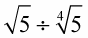##### Linear Algebra For Dummies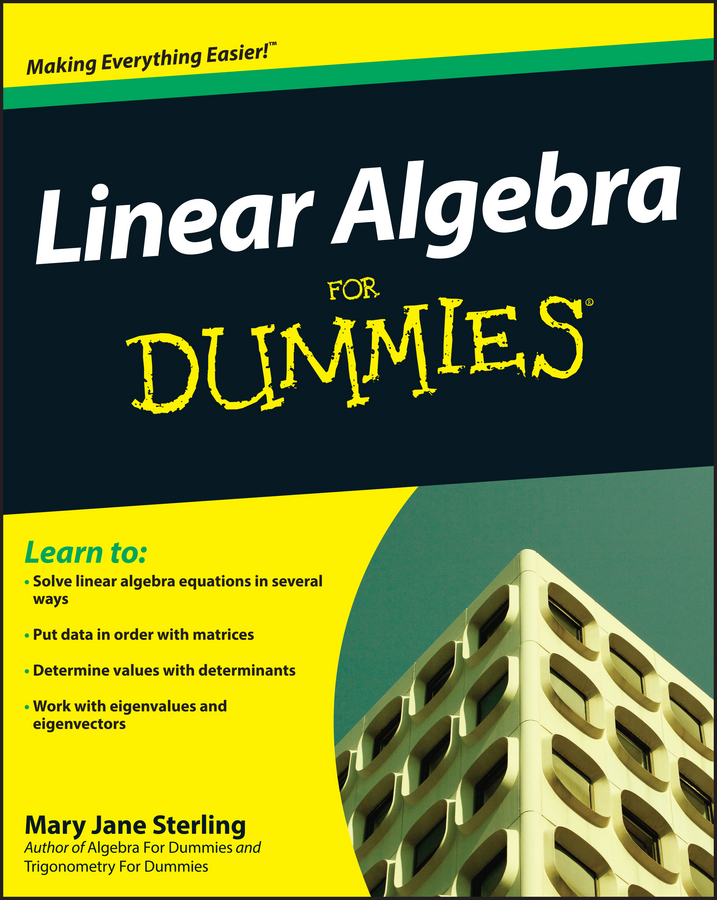Square roots, which use the radical symbol, are nonbinary operations — operations which involve just one number — that ask you, “What number times itself gives you this number under the radical?” Finding square roots is a relatively common operation in algebra, but working with and combining the roots isn’t always so clear.

Expressions with radicals can be multiplied or divided as long as the root power or value under the radical is the same. Expressions with radicals cannot be added or subtracted unless both the root power and the value under the radical are the same.

When you find square roots, the symbol for that operation is called a radical. The root power refers to the number outside and to the upper left of the radical. If there is no number, you assume that the root power is 2.

When it comes to combining radicals, there are just a couple of simple rules to remember:

• Addition and subtraction can be performed if the root power and the value under the radical are the same.

Examples:

These radicals cannot be combined because the operation is addition, and the value under the radical is not the same: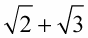These radicals can be combined because the root power and the numbers under the radical are the same:These radicals cannot be combined because the operation is subtraction, and the root power isn’t the same: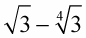• Multiplication and division can be performed if the root powers are the same.

Examples:

These radicals can be combined because the operation is multiplication, and the root power is the same: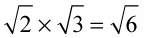These radicals can be combined because the operation is division, and the root power is the same: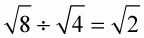These radicals cannot be combined because the operation is division, and the root power isn’t the same: